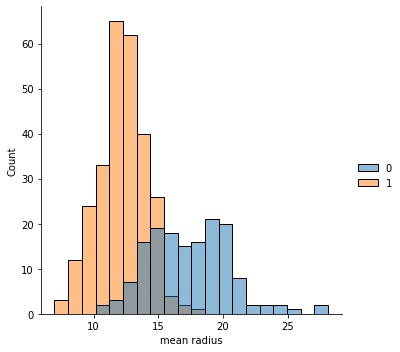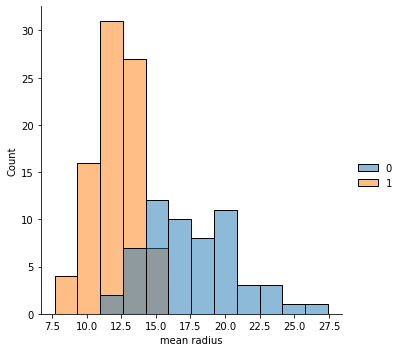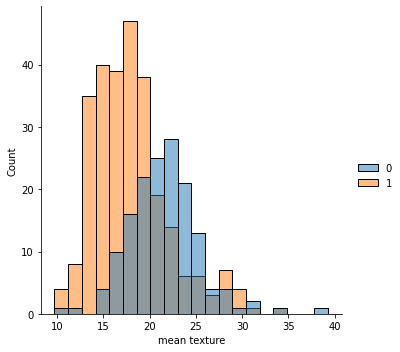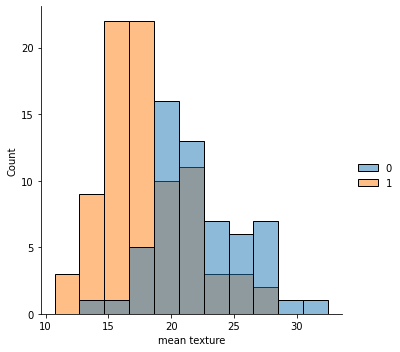# Scikit-Learn Model Card Toolkit Demo

## Background

This notebook demonstrates how to generate a model card using the Model Card Toolkit with a scikit-learn model in a Jupyter/Colab environment. You can learn more about model cards at https://modelcards.withgoogle.com/about.

## Setup

We first need to install and import the necessary packages.

### Upgrade to Pip 20.2 and Install Packages

````pip install -q --upgrade pip==20.2`
`pip install -q -U seaborn scikit-learn model-card-toolkit`
```

### Did you restart the runtime?

If you are using Google Colab, the first time that you run the cell above, you must restart the runtime (Runtime > Restart runtime ...).

### Import packages

We import necessary packages, including scikit-learn.

``````from datetime import date
from io import BytesIO
from IPython import display
from model_card_toolkit import ModelCardToolkit
from sklearn.model_selection import train_test_split
from sklearn.metrics import plot_roc_curve, plot_confusion_matrix

import base64
import matplotlib.pyplot as plt
import pandas as pd
import seaborn as sns
import uuid
``````

This example uses the Breast Cancer Wisconsin Diagnostic dataset that scikit-learn can load using the load_breast_cancer() function.

``````cancer = load_breast_cancer()

X = pd.DataFrame(cancer.data, columns=cancer.feature_names)
y = pd.Series(cancer.target)

X_train, X_test, y_train, y_test = train_test_split(X, y)
``````
``````X_train.head()
``````
``````y_train.head()
``````
```28     0
157    1
381    1
436    1
71     1
dtype: int64
```

## Plot data

We will create several plots from the data that we will include in the model card.

``````# Utility function that will export a plot to a base-64 encoded string that the model card will accept.

def plot_to_str():
img = BytesIO()
plt.savefig(img, format='png')
return base64.encodebytes(img.getvalue()).decode('utf-8')
``````
``````# Plot the mean radius feature for both the train and test sets

````````````# Plot the mean texture feature for both the train and test sets

sns.displot(x=X_train['mean texture'], hue=y_train)
mean_texture_train = plot_to_str()

sns.displot(x=X_test['mean texture'], hue=y_test)
mean_texture_test = plot_to_str()
``````## Train model

``````# Create a classifier and fit the training data

``````

## Evaluate model

``````# Plot a ROC curve

plot_roc_curve(clf, X_test, y_test)
roc_curve = plot_to_str()
````````````# Plot a confusion matrix

plot_confusion_matrix(clf, X_test, y_test)
confusion_matrix = plot_to_str()
``````## Create a model card

### Initialize toolkit and model card

``````mct = ModelCardToolkit()

model_card = mct.scaffold_assets()
``````

### Annotate information into model card

``````model_card.model_details.name = 'Breast Cancer Wisconsin (Diagnostic) Dataset'
model_card.model_details.overview = (
'This model predicts whether breast cancer is benign or malignant based on '
'image measurements.')
model_card.model_details.owners = [
{'name': 'Model Cards Team', 'contact': 'model-cards@google.com'}
]
model_card.model_details.references = [
'https://archive.ics.uci.edu/ml/datasets/Breast+Cancer+Wisconsin+(Diagnostic)',
'https://minds.wisconsin.edu/bitstream/handle/1793/59692/TR1131.pdf'
]
model_card.model_details.version.name = str(uuid.uuid4())
model_card.model_details.version.date = str(date.today())

model_card.considerations.ethical_considerations = [{
'name': ('Manual selection of image sections to digitize could create '
'selection bias'),
'mitigation_strategy': 'Automate the selection process'
}]
model_card.considerations.limitations = ['Breast cancer diagnosis']
model_card.considerations.use_cases = ['Breast cancer diagnosis']
model_card.considerations.users = ['Medical professionals', 'ML researchers']

model_card.model_parameters.data.train.graphics.description = (
f'{len(X_train)} rows with {len(X_train.columns)} features')
model_card.model_parameters.data.train.graphics.collection = [
{'image': mean_texture_train}
]
model_card.model_parameters.data.eval.graphics.description = (
f'{len(X_test)} rows with {len(X_test.columns)} features')
model_card.model_parameters.data.eval.graphics.collection = [
{'image': mean_texture_test}
]
model_card.quantitative_analysis.graphics.description = (
'ROC curve and confusion matrix')
model_card.quantitative_analysis.graphics.collection = [
{'image': roc_curve},
{'image': confusion_matrix}
]

mct.update_model_card_json(model_card)
``````

## Generate model card

``````# Return the model card document as an HTML page

html = mct.export_format()

display.display(display.HTML(html))
``````
[{ "type": "thumb-down", "id": "missingTheInformationINeed", "label":"Missing the information I need" },{ "type": "thumb-down", "id": "tooComplicatedTooManySteps", "label":"Too complicated / too many steps" },{ "type": "thumb-down", "id": "outOfDate", "label":"Out of date" },{ "type": "thumb-down", "id": "samplesCodeIssue", "label":"Samples / code issue" },{ "type": "thumb-down", "id": "otherDown", "label":"Other" }]
[{ "type": "thumb-up", "id": "easyToUnderstand", "label":"Easy to understand" },{ "type": "thumb-up", "id": "solvedMyProblem", "label":"Solved my problem" },{ "type": "thumb-up", "id": "otherUp", "label":"Other" }]